# 六、非刚性人脸跟踪

• 概述：本节介绍面部跟踪的简要历史。
• 工具：本节概述了本章中使用的通用结构和约定。 它包括面向对象的设计，数据存储和表示，以及用于数据收集和标注的工具。
• 几何约束：本节描述如何从训练数据中学习面部几何及其变化，并在跟踪过程中利用面部几何约束其解决方案。 这包括将人脸建模为线性形状模型，以及如何将全局转换集成到其表示中。
• 人脸特征检测器：本节介绍如何学习人脸特征的外观，以便在要跟踪面部的图像中检测人脸特征。
• 人脸检测和初始化：本节介绍如何使用人脸检测初始化跟踪过程。
• 人脸跟踪：本节通过图像对齐过程将前面描述的所有组件组合到跟踪系统中。 还讨论了可以期望系统最佳工作的设置。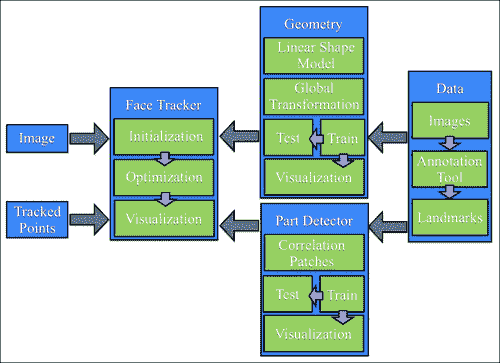# 工具

## 面向对象设计

#include <opencv2/opencv.hpp>
using namespace cv;
class foo{
public:
Mat a;
type_b b;
void write(FileStorage &fs) const{
assert(fs.isOpened());
fs << "{" << "a"  << a << "b"  << b << "}";
}
assert(node.type() == FileNode::MAP);
node["a"] >> a; node["b"] >> b;
}
};


void write(FileStorage& fs, const string&, const foo& x){
x.write(fs);
}
void read(const FileNode& node, foo& x,const foo& default){
}


template <class T>
f["ft object"] >> x; f.release(); return x;
}
template<class T>
void save_ft(const char* fname,const T& x){
FileStorage f(fname,FileStorage::WRITE);
f << "ft object" << x; f.release();
}


#include "opencv_hotshots/ft/ft.hpp"
#include "foo.hpp"
int main(){
...
foo A; save_ft<foo>("foo.xml",A);
...
...
}


## 数据收集：图像和视频标注

### 训练数据类型

• 图像：该组件是包含整个面部的图像（静止图像或视频帧）的集合。 为了获得最佳结果，此集合应专门针对随后部署跟踪器的条件类型（即身份，照明，与摄像机的距离，捕获设备等）。 同样重要的是，集合中的面孔必须具有预期应用期望的各种头部姿势和面部表情。
• 标注：该组件在每个图像中按顺序排列了手工标记的位置，这些位置与要跟踪的每个人脸特征相对应。 更多的人脸特征通常会导致跟踪器更强大，因为跟踪算法可以使用它们的测量值来相互增强。 常见跟踪算法的计算成本通常与人脸特征的数量成线性比例。
• 对称性索引：该组件具有每个人脸特征点的索引，这些索引定义了其双边对称特征。 这可以用来镜像训练图像，有效地使训练集大小加倍，并使数据沿 y 轴对称。
• 连接性索引：该组件具有一组标注的索引对，这些标注对定义了人脸特征的语义解释。 这些连接对于可视化跟踪结果很有用。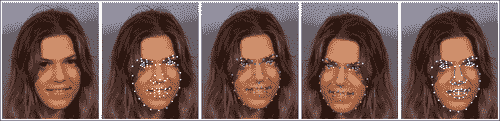class ft_data{
public:
vector<int> symmetry;
vector<Vec2i> connections;
vector<string> imnames;
vector<vector<Point2f> > points;
…
}


Vec2iPoint2f类型分别是两个整数和 2D 浮点坐标的向量的 OpenCV 类。 symmetry向量具有与面部上的特征点一样多的成分（由用户定义）。 connections中的每一个都定义了一个连接人脸特征的从零开始的索引对。 由于训练集可能非常大，而不是直接存储图像，因此该类将每个图像的文件名存储在imnames成员变量中（请注意，这要求图像必须位于文件名的相同相对路径中，来保持有效）。 最后，对于每个训练图像，将人脸特征位置的集合作为浮点坐标的向量存储在points成员变量中。

ft_data类实现了许多用于访问数据的便捷方法。 要访问数据集中的图像，get_image函数将图像加载到指定索引idx，并可选地围绕 y 轴进行镜像，如下所示：

Mat
ft_data::get_image(
const int idx,   //index of image to load from file
const int flag){ //0=gray,1=gray+flip,2=rgb,3=rgb+flip
if((idx < 0) || (idx >= (int)imnames.size()))return Mat();
Mat img,im;
if(flag % 2 != 0)flip(img,im,1); else im = img;
return im;
}


vector<Point2f>
ft_data::get_points(
const int idx,       //index of image corresponding to pointsconst bool flipped){ //is the image flipped around the y-axis?
if((idx < 0) || (idx >= (int)imnames.size()))
return vector<Point2f>();
vector<Point2f> p = points[idx];
if(flipped){
Mat im = this->get_image(idx,0); int n = p.size();
vector<Point2f> q(n);
for(int i = 0; i < n; i++){
q[i].x = im.cols-1-p[symmetry[i]].x;
q[i].y = p[symmetry[i]].y;
}return q;
}else return p;
}


void
ft_data::rm_incomplete_samples(){
int n = points.size(),N = points.size();
for(int i = 1; i < N; i++)n = max(n,int(points[i].size()));
for(int i = 0; i < int(points.size()); i++){
if(int(points[i].size()) != n){
points.erase(points.begin()+i);
imnames.erase(imnames.begin()+i); i--;
}else{
int j = 0;
for(; j < n; j++){
if((points[i][j].x <= 0) ||
(points[i][j].y <= 0))break;
}
if(j < n){
points.erase(points.begin()+i);
imnames.erase(imnames.begin()+i); i--;
}
}
}
}


ft_data类实现了序列化函数readwrite，因此可以轻松存储和加载。 例如，保存数据集可以很简单地完成：

ft_data D;                        //instantiate data structure
…                                 //populate data
save_ft<ft_data>("mydata.xml",D); //save data


### 标注工具

1. 捕获图像：在第一步中，图像流显示在屏幕上，并且用户可以通过按S键选择要标注的图像。 最好的标注特征集是最大程度地扩展了面部跟踪系统将要跟踪的面部行为范围的那些特征。
2. 标注第一张图像：在此第二步中，向用户呈现在上一阶段中选择的第一张图像。 然后，用户继续在与需要跟踪的人脸特征有关的位置上单击图像。
3. 标注连接：在此第三步中，为了更好地可视化形状，需要定义点的连接结构。 在此，向用户显示与上一阶段相同的图像，其中现在的任务是依次单击一组点对，以建立人脸模型的连接结构。
4. 标注对称性：在此步骤中，仍然使用相同的图像，用户选择显示双边对称性的点对。
5. 标注剩余图像：在此最后一步中，此处的过程与步骤 2 相似，不同之处在于用户可以浏览图像集并异步标注它们。

### 预标注的数据（MUCT 数据集）

1. 下载图像集：在此步骤中，可以通过将文件muct-a-jpg-v1.tar.gz下载到muct-e-jpg-v1.tar.gz并解压缩来获取数据集中的所有图像。 这将生成一个新文件夹，其中将存储所有图像。
2. 下载标注：在此步骤中，下载包含标注muct-landmarks-v1.tar.gz的文件。 将该文件保存并解压缩到与下载图像相同的文件夹中。
3. 使用标注工具定义连接和对称性：在此步骤中，从命令行发出命令./annotate -m $mdir -d$odir，其中$mdir表示保存 MUCT 数据集的文件夹，$odir表示将annotations.yaml文件（包含作为ft_data对象存储的数据）写入到的文件夹。

# 几何约束

class shape_model{ //2d linear shape model
public:
Mat p; //parameter vector (kx1) CV_32F
Mat V; //linear subspace (2nxk) CV_32F
Mat e; //parameter variance (kx1) CV_32F
Mat C; //connectivity (cx2) CV_32S
...
void calc_params(
const vector<Point2f> &pts,  //points to compute parameters
const Mat &weight = Mat(),    //weight/point (nx1) CV_32F
const float c_factor = 3.0); //clamping factor
...
vector<Point2f>              //shape described by parameters
calc_shape();
...
void train(
const vector<vector<Point2f> > &p, //N-example shapes
const vector<Vec2i> &con = vector<Vec2i>(),//connectivity
const float frac = 0.95, //fraction of variation to retain
const int kmax = 10);   //maximum number of modes to retain
...
}


## 普氏分析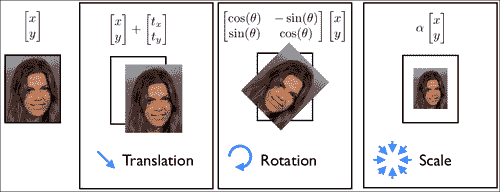#define fl at<float>
Mat shape_model::procrustes(
const Mat &X,       //interleaved raw shape data as columns
const int itol,     //maximum number of iterations to try
const float ftol)   //convergence tolerance
{
int N = X.cols,n = X.rows/2; Mat Co,P = X.clone();//copy
for(int i = 0; i < N; i++){
Mat p = P.col(i);            //i'th shape
float mx = 0,my = 0;         //compute centre of mass...
for(int j = 0; j < n; j++){  //for x and y separately
mx += p.fl(2*j); my += p.fl(2*j+1);
}
mx /= n; my /= n;
for(int j = 0; j < n; j++){ //remove center of mass
p.fl(2*j) -= mx; p.fl(2*j+1) -= my;
}
}
for(int iter = 0; iter < itol; iter++){
Mat C = P*Mat::ones(N,1,CV_32F)/N; //compute normalized...
normalize(C,C);                    //canonical shape
if(iter > 0){if(norm(C,Co) < ftol)break;} //converged?
Co = C.clone();               //remember current estimate
for(int i = 0; i < N; i++){
Mat R = this->rot_scale_align(P.col(i),C);
for(int j = 0; j < n; j++){ //apply similarity transform
float x = P.fl(2*j,i),y = P.fl(2*j+1,i);
P.fl(2*j  ,i) = R.fl(0,0)*x + R.fl(0,1)*y;
P.fl(2*j+1,i) = R.fl(1,0)*x + R.fl(1,1)*y;
}
}
}return P; //returned procrustes aligned shapes
}


Mat shape_model::rot_scale_align(
const Mat &src, //[x1;y1;...;xn;yn] vector of source shape
const Mat &dst) //destination shape
{
//construct linear system
int n = src.rows/2; float a=0,b=0,d=0;
for(int i = 0; i < n; i++){
d+= src.fl(2*i)*src.fl(2*i  )+src.fl(2*i+1)*src.fl(2*i+1);
a+= src.fl(2*i)*dst.fl(2*i  )+src.fl(2*i+1)*dst.fl(2*i+1);
b+= src.fl(2*i)*dst.fl(2*i+1)-src.fl(2*i+1)*dst.fl(2*i  );
}
a /= d; b /= d;//solve linear system
return (Mat_<float>(2,2) << a,-b,b,a);
}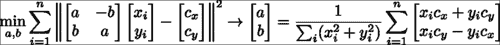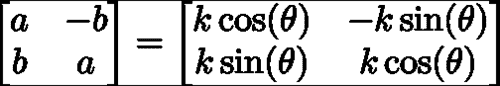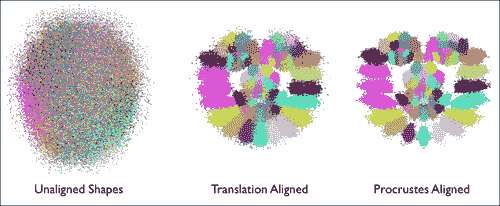## 线性形状模型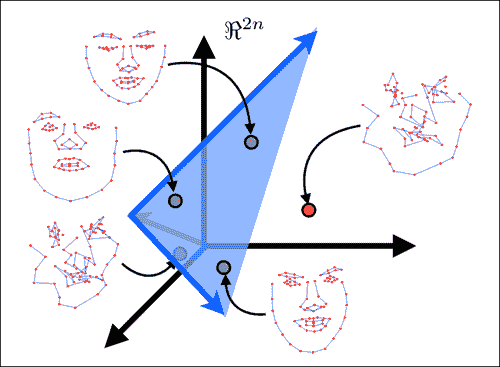SVD svd(dY*dY.t());
int m = min(min(kmax,N-1),n-1);
float vsum = 0; for(int i = 0; i < m; i++)vsum += svd.w.fl(i);
float v = 0; int k = 0;
for(k = 0; k < m; k++){
v += svd.w.fl(k); if(v/vsum >= frac){k++; break;}
}
if(k > m)k = m;
Mat D = svd.u(Rect(0,0,k,2*n));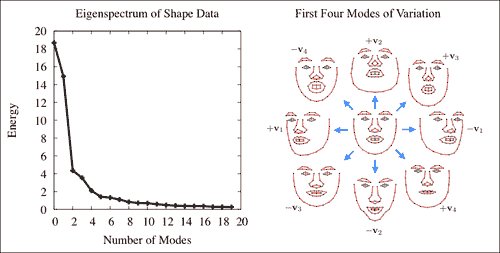## 组合的局部-全局表示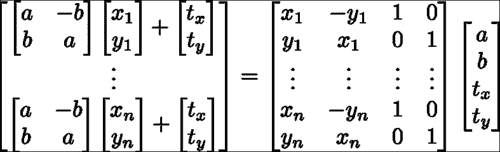shape_model类中，此子空间是使用calc_rigid_basis函数生成的。 从中生成子空间的形状（即前面方程中的xy分量）是 Procustes 对齐的形状（即规范形状）的平均形状。 除了以上述形式构造子空间外，矩阵的每一列都被标准化为单位长度。 在shape_model::train函数中，上一节中描述的变量dY通过投影与刚性运动有关的数据分量来计算，如下所示：

Mat R = this->calc_rigid_basis(Y); //compute rigid subspace
Mat P = R.t()*Y; Mat dY = Y – R*P; //project-out rigidity


V.create(2*n,4+k,CV_32F);                  //combined subspace
Mat Vr = V(Rect(0,0,4,2*n)); R.copyTo(Vr); //rigid subspace
Mat Vd = V(Rect(4,0,k,2*n)); D.copyTo(Vd); //nonrigid subspace


p = V.t()*s;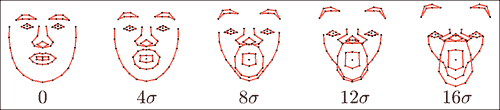Mat Q = V.t()*X;            //project raw data onto subspace
for(int i = 0; i < N; i++){ //normalize coordinates w.r.t scale
float v = Q.fl(0,i); Mat q = Q.col(i); q /= v;
}
e.create(4+k,1,CV_32F); multiply(Q,Q,Q);
for(int i = 0; i < 4+k; i++){
if(i < 4)e.fl(i) = -1; //no clamping for rigid coefficients
else e.fl(i) = Q.row(i).dot(Mat::ones(1,N,CV_32F))/(N-1);
}


void shape_model::clamp(
const float c){ //clamping as fraction of standard deviation
double scale = p.fl(0);        //extract scale
for(int i = 0; i < e.rows; i++){
if(e.fl(i) < 0)continue;     //ignore rigid components
float v = c*sqrt(e.fl(i));   //c*standard deviations box
if(fabs(p.fl(i)/scale) > v){ //preserve sign of coordinate
if(p.fl(i) > 0)p.fl(i) =  v*scale; //positive threshold
else p.fl(i) = -v*scale; //negative threshold
}
}
}


## 训练和可视化

train_shape_model.cpp中可以找到用于从标注数据中训练形状模型的示例程序。 在命令行参数argv包含标注数据的路径的情况下，训练首先将数据加载到内存中并删除不完整的样本，如下所示：

ft_data data = load_ft<ft_data>(argv);
data.rm_incomplete_samples();


vector<vector<Point2f> > points;
for(int i = 0; i < int(data.points.size()); i++){
points.push_back(data.get_points(i,false));
if(mirror)points.push_back(data.get_points(i,true));
}


shape_model smodel; smodel.train(points,data.connections,frac,kmax);


save_ft(argv,smodel);


shape_model smodel = load_ft<shape_model>(argv);


int n = smodel.V.rows/2;
float scale = calc_scale(smodel.V.col(0),200);
float tranx =
n*150.0/smodel.V.col(2).dot(Mat::ones(2*n,1,CV_32F));
float trany =
n*150.0/smodel.V.col(3).dot(Mat::ones(2*n,1,CV_32F));


### 注意

vector<float> val;
for(int i = 0; i < 50; i++)val.push_back(float(i)/50);
for(int i = 0; i < 50; i++)val.push_back(float(50-i)/50);
for(int i = 0; i < 50; i++)val.push_back(-float(i)/50);
for(int i = 0; i < 50; i++)val.push_back(-float(50-i)/50);


  Mat img(300,300,CV_8UC3); namedWindow("shape model");
while(1){
for(int k = 4; k < smodel.V.cols; k++){
for(int j = 0; j < int(val.size()); j++){
Mat p = Mat::zeros(smodel.V.cols,1,CV_32F);
p.at<float>(0) = scale;
p.at<float>(2) = tranx;
p.at<float>(3) = trany;
p.at<float>(k) = scale*val[j]3.0
sqrt(smodel.e.at<float>(k));
p.copyTo(smodel.p); img = Scalar::all(255);
vector<Point2f> q = smodel.calc_shape();
draw_shape(img,q,smodel.C);
imshow("shape model",img);
if(waitKey(10) == 'q')return 0;
}
}
}


# 人脸特征检测器

• 精确度与鲁棒性：在一般物体检测中，目的是找到图像中物体的粗略位置。 人脸特征检测器需要对特征位置进行高度精确的估计。 几个像素的误差在对象检测中被认为是无关紧要的，但这可能意味着通过特征检测在面部表情估计中的微笑和皱眉之间的差异。
• 有限的支持空间带来的歧义：通常假设通用对象检测中的关注对象具有足够的图像结构，因此可以可靠地将其与不包含该对象的图像区域区分开。 对于通常具有有限空间支持的人脸特征通常不是这种情况。 这是因为不包含对象的图像区域通常会显示出与人脸特征非常相似的结构。 例如，从以特征为中心的小边界框看，脸部外围的特征可以很容易地与其他任何包含穿过其中心的强边缘的图像块混淆。
• 计算复杂度：通用对象检测旨在查找图像中对象的所有实例。 另一方面，脸部追踪需要所有脸部特征的位置，通常范围从 20 到 100 个左右。 因此，有效地评估每个特征检测器的能力对于构建可以实时运行的面部跟踪器至关重要。

class patch_model{
public:
Mat P; //normalized patch
...
Mat                          //response map
calc_response(
const Mat &im,               //image patch of search region
const bool sum2one = false); //normalize to sum-to-one?
...
void
train(const vector<Mat> &images, //training image patches
const Size psize,                //patch size
const float var = 1.0,           //ideal response variance
const float lambda = 1e-6,       //regularization weight
const float mu_init = 1e-3,      //initial step size
const int nsamples = 1000,       //number of samples
const bool visi = false);        //visualize process?
...
};


## 基于相关性的补丁模型

### 学习判别式补丁模型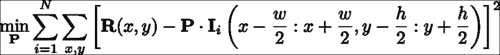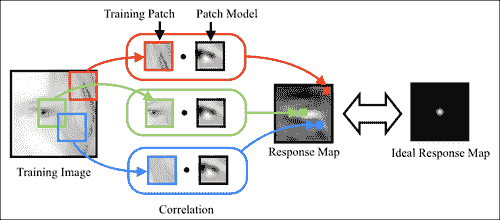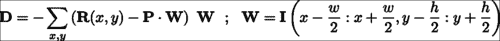patch_model类中，此学习过程是在train函数中实现的：

void
patch_model::train(
const vector<Mat> &images, //featured centered training images
const Size psize,          //desired patch model size
const float var,           //variance of annotation error
const float lambda,        //regularization parameter
const float mu_init,       //initial step size
const int nsamples,        //number of stochastic samples
const bool visi){          //visualise training process
int N = images.size(),n = psize.width*psize.height;
int dx = wsize.width-psize.width;   //center of response map
int dy = wsize.height-psize.height; //...
Mat F(dy,dx,CV_32F); //ideal response map
for(int y = 0; y < dy; y++){  float vy = (dy-1)/2 - y;
for(int x = 0; x < dx; x++){float vx = (dx-1)/2 - x;
F.fl(y,x) = exp(-0.5*(vx*vx+vy*vy)/var); //Gaussian
}
}
normalize(F,F,0,1,NORM_MINMAX); //normalize to [0:1] range

//allocate memory
Mat I(wsize.height,wsize.width,CV_32F);
Mat dP(psize.height,psize.width,CV_32F);
Mat O = Mat::ones(psize.height,psize.width,CV_32F)/n;
P = Mat::zeros(psize.height,psize.width,CV_32F);

RNG rn(getTickCount()); //random number generator
double mu=mu_init,step=pow(1e-8/mu_init,1.0/nsamples);
for(int sample = 0; sample < nsamples; sample++){
int i = rn.uniform(0,N); //randomly sample image index
I = this->convert_image(images[i]); dP = 0.0;
for(int y = 0; y < dy; y++){ //compute stochastic gradient
for(int x = 0; x < dx; x++){
Mat Wi=I(Rect(x,y,psize.width,psize.height)).clone();
Wi -= Wi.dot(O); normalize(Wi,Wi); //normalize
dP += (F.fl(y,x) – P.dot(Wi))*Wi;
}
}
P += mu*(dP - lambda*P); //take a small step
mu *= step;              //reduce step size
...
}return;
}


I += 1.0; log(I,I);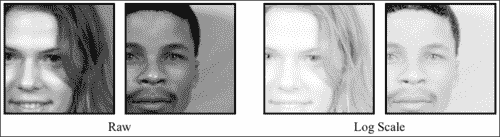### 生成式与判别式补丁模型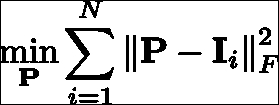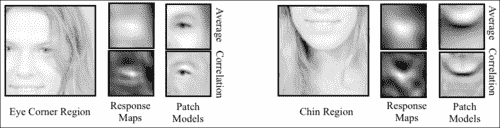## 解释整体几何变换

patch_models类存储每个人脸特征的相关补丁模型以及在其中训练它们的参考框架。 面部跟踪器代码直接与patch_models类（而不是patch_model类）联系以获取特征检测。 此类声明的以下代码片段突出了其主要功能：

class patch_models{
public:
Mat reference;      //reference shape [x1;y1;...;xn;yn]
vector<patch_model> patches; //patch model/facial feature
...
void
train(ft_data &data,        //annotated image and shape data
const vector<Point2f> &ref, //reference shape
const Size psize,           //desired patch size
const Size ssize,           //training search window size
const bool mirror = false,  //use mirrored training data
const float var = 1.0,      //variance of annotation error
const float lambda = 1e-6,  //regularisation weight
const float mu_init = 1e-3, //initial step size
const int nsamples = 1000,  //number of samples
const bool visi = false);   //visualise training procedure?
...
vector<Point2f>//location of peak responses/feature in image
calc_peaks(
const Mat &im,    //image to detect features in
const vector<Point2f> &points, //current estimate of shape
const Size ssize = Size(21,21)); //search window size
...
};


reference形状存储为(x, y)坐标的交错集，用于标准化训练图像的比例和旋转，以及随后在部署测试图像时对其进行标准化。 在patch_models::train函数中，这首先通过使用patch_models::calc_simil函数计算给定图像的reference形状和带标注的形状之间的相似度变换来解决，这解决了与shape_model::procrustes函数相似的问题 ，尽管只有一对形状。 由于旋转和缩放在所有人脸特征上都是通用的，因此图像标准化过程仅需要调整此相似度变换，以解决图像中每个特征的中心和标准化图像补丁的中心。 在patch_models::train中，实现方式如下：

Mat S = this->calc_simil(pt),A(2,3,CV_32F);
A.fl(0,0) = S.fl(0,0); A.fl(0,1) = S.fl(0,1);
A.fl(1,0) = S.fl(1,0); A.fl(1,1) = S.fl(1,1);
A.fl(0,2) = pt.fl(2*i  ) - (A.fl(0,0)*(wsize.width -1)/2 +
A.fl(0,1)*(wsize.height-1)/2);
A.fl(1,2) = pt.fl(2*i+1) – (A.fl(1,0)*(wsize.width -1)/2 +
A.fl(1,1)*(wsize.height-1)/2);
Mat I; warpAffine(im,I,A,wsize,INTER_LINEAR+WARP_INVERSE_MAP);


vector<Point2f>
patch_models::calc_peaks(const Mat &im,
const vector<Point2f> &points,const Size ssize){
int n = points.size(); assert(n == int(patches.size()));
Mat pt = Mat(points).reshape(1,2*n);
Mat S = this->calc_simil(pt);
Mat Si = this->inv_simil(S);
vector<Point2f> pts = this->apply_simil(Si,points);
for(int i = 0; i < n; i++){
Size wsize = ssize + patches[i].patch_size();
Mat A(2,3,CV_32F),I;
A.fl(0,0) = S.fl(0,0); A.fl(0,1) = S.fl(0,1);
A.fl(1,0) = S.fl(1,0); A.fl(1,1) = S.fl(1,1);
A.fl(0,2) = pt.fl(2*i  ) - (A.fl(0,0)*(wsize.width -1)/2 +
A.fl(0,1)*(wsize.height-1)/2);
A.fl(1,2) = pt.fl(2*i+1) – (A.fl(1,0)*(wsize.width -1)/2 +
A.fl(1,1)*(wsize.height-1)/2);
warpAffine(im,I,A,wsize,INTER_LINEAR+WARP_INVERSE_MAP);
Mat R = patches[i].calc_response(I,false);
Point maxLoc; minMaxLoc(R,0,0,0,&maxLoc);
pts[i] = Point2f(pts[i].x + maxLoc.x - 0.5*ssize.width,
pts[i].y + maxLoc.y - 0.5*ssize.height);
}return this->apply_simil(S,pts);



## 训练和可视化

train_patch_model.cpp中可以找到用于从标注数据中训练补丁模型的示例程序。 在命令行参数argv包含标注数据的路径的情况下，训练首先将数据加载到内存中并删除不完整的样本：

ft_data data = load_ft<ft_data>(argv);
data.rm_incomplete_samples();


shape_model smodel = load_ft<shape_model>(argv);


smodel.p = Scalar::all(0.0);
smodel.p.fl(0) = calc_scale(smodel.V.col(0),width);
vector<Point2f> r = smodel.calc_shape();


calc_scale函数计算比例因子，以将平均形状（即shape_model::V的第一列）转换为宽度为width的形状。 定义参考形状r 后，可以通过单个函数调用来训练补丁模型集：

patch_models pmodel; pmodel.train(data,r,Size(psize,psize),Size(ssize,ssize));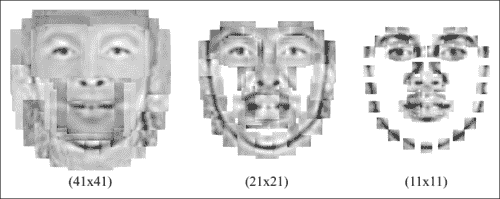# 人脸检测和初始化

face_detector类完全实现了此解决方案。 声明其功能的摘录如下：

class face_detector{ //face detector for initialisation
public:
string detector_fname; //file containing cascade classifier
Vec3f detector_offset; //offset from center of detection
Mat reference;         //reference shape

vector<Point2f>  //points describing detected face in image
detect(const Mat &im,          //image containing face
const float scaleFactor = 1.1,//scale increment
const int minNeighbours = 2,  //minimum neighborhood size
const Size minSize = Size(30,30));//minimum window size

void
train(ft_data &data,               //training data
const Mat &ref,                    //reference shape
const bool mirror = false,         //mirror data?
const bool visi = false,           //visualize training?
const float frac = 0.8, //fraction of points in detection
const float scaleFactor = 1.1, //scale increment
const int minNeighbours = 2,   //minimum neighbourhood size
const Size minSize = Size(30,30)); //minimum window size
...
};


Mat gray; //convert image to grayscale and histogram equalize
if(im.channels() == 1)gray = im;
else cvtColor(im,gray,CV_RGB2GRAY);
Mat eqIm; equalizeHist(gray,eqIm);
vector<Rect> faces; //detect largest face in image
detector.detectMultiScale(eqIm,faces,scaleFactor,
minNeighbours,0
|CV_HAAR_FIND_BIGGEST_OBJECT
|CV_HAAR_SCALE_IMAGE,minSize);
if(faces.size() < 1){return vector<Point2f>();}

Rect R = faces; Vec3f scale = detector_offset*R.width;
int n = reference.rows/2; vector<Point2f> p(n);
for(int i = 0; i < n; i++){ //predict face placement
p[i].x = scale*reference.fl(2*i  ) +
R.x + 0.5 * R.width  + scale;
p[i].y = scale*reference.fl(2*i+1) +
R.y + 0.5 * R.height + scale;
}return p;



detector.load(fname.c_str()); detector_fname = fname; reference = ref.clone();


patch_models类中的参考形状一样，参考形状的方便选择是数据集中的标准化平均脸部形状。 然后，将cv::CascadeClassifier应用于数据集中的每个图像（以及可选的镜像副本），并检查结果以确保足够的带标注的点位于检测到的边界框中（请参见本节末尾的图） 防止因误检而学习：

if(this->enough_bounded_points(pt,faces,frac)){
Point2f center = this->center_of_mass(pt);
float w = faces.width;
xoffset.push_back((center.x -
(faces.x+0.5*faces.width ))/w);
yoffset.push_back((center.y -
(faces.y+0.5*faces.height))/w);
zoffset.push_back(this->calc_scale(pt)/w);
}


Mat X = Mat(xoffset),Xsort,Y = Mat(yoffset),Ysort,Z = Mat(zoffset),Zsort;
cv::sort(X,Xsort,CV_SORT_EVERY_COLUMN|CV_SORT_ASCENDING);
int nx = Xsort.rows;
cv::sort(Y,Ysort,CV_SORT_EVERY_COLUMN|CV_SORT_ASCENDING);
int ny = Ysort.rows;
cv::sort(Z,Zsort,CV_SORT_EVERY_COLUMN|CV_SORT_ASCENDING);
int nz = Zsort.rows;
detector_offset =
Vec3f(Xsort.fl(nx/2),Ysort.fl(ny/2),Zsort.fl(nz/2));


ft_data data = load_ft<ft_data>(argv);
smodel.set_identity_params();
vector<Point2f> r = smodel.calc_shape();
Mat ref = Mat(r).reshape(1,2*r.size());


face_detector detector;
detector.train(data,argv,ref,mirror,true,frac);
save_ft<face_detector>(argv,detector);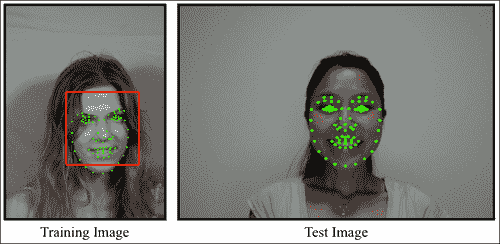# 人脸追踪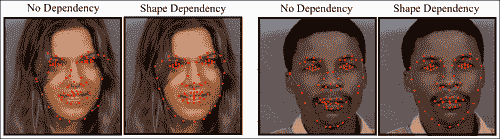## 人脸追踪器的实现

class face_tracker{
public:
bool tracking;          //are we in tracking mode?
fps_timer timer;        //frames/second timer
vector<Point2f> points; //current tracked points
face_detector detector; //detector for initialisation
shape_model smodel;     //shape model
patch_models pmodel;    //feature detectors

face_tracker(){tracking = false;}

int                             //0 = failure
track(const Mat &im,            //image containing face
const face_tracker_params &p =  //fitting parameters
face_tracker_params());     //default tracking parameters

void
reset(){                            //reset tracker
tracking = false; timer.reset();
}
...
protected:
...
vector<Point2f>   //points for fitted face in image
fit(const Mat &image,//image containing face
const vector<Point2f> &init,   //initial point estimates
const Size ssize = Size(21,21),//search region size
const bool robust = false,     //use robust fitting?
const int itol = 10,    //maximum number of iterations
const float ftol = 1e-3);      //convergence tolerance
};


int face_tracker::
track(const Mat &im,const face_tracker_params &p){
Mat gray; //convert image to grayscale
if(im.channels()==1)gray=im;
else cvtColor(im,gray,CV_RGB2GRAY);
if(!tracking) //initialize
points = detector.detect(gray,p.scaleFactor,
p.minNeighbours,p.minSize);
if((int)points.size() != smodel.npts())return 0;
for(int level = 0; level < int(p.ssize.size()); level++)
points = this->fit(gray,points,p.ssize[level],
p.robust,p.itol,p.ftol);
tracking = true; timer.increment();  return 1;
}


smodel.calc_params(init);
vector<Point2f> pts = smodel.calc_shape();
vector<Point2f> peaks = pmodel.calc_peaks(image,pts,ssize);


smodel.calc_params(peaks);
pts = smodel.calc_shape();


## 训练和可视化

face_tracker tracker;
save_ft<face_tracker>(argv,tracker);


## 通用模型与个人模型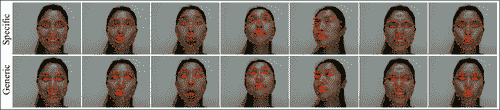# 参考

• Procrustes Problems, Gower, John C. and Dijksterhuis, Garmt B, Oxford University Press, 2004.